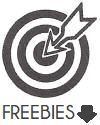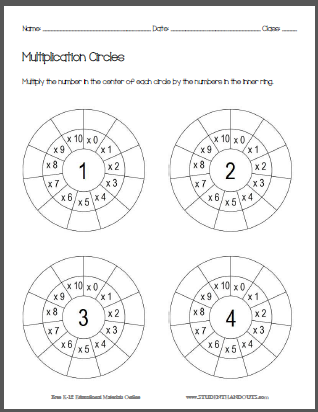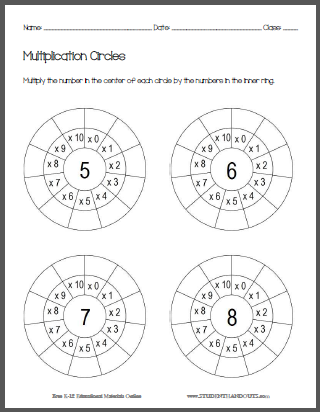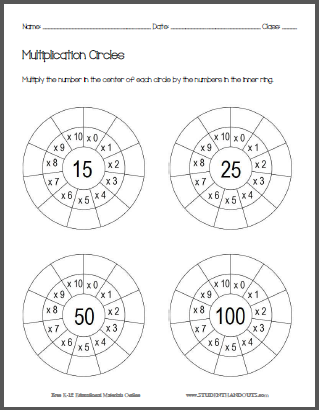Multiplication Circle Worksheets | Student Handouts
 Share the learning joy!

Multiplication Circle Worksheets
 Free to Print (PDF Files) - Grade 3 > Grade 3 Math > Grade 3 Operations and Algebraic ThinkingThe basic worksheet above features a large multiplication circle. A number is placed in the center of the multiplication circle (the teacher writes a number before copying a class set, or the student draws a number from a jar). In the outer ring of blank spaces, the student puts the result of multiplying the center number by the number in the inner ring. This large sheet is very handy for introducing the concept, and displays nicely on a Smartboard. Click here to print. Look below for more.

The worksheets below are for individual practice. They also make a handy binder reference tool once correctly completed.This worksheet has students multiply one through four by zero through ten. Click here to print.
This worksheet has students multiply five through eight by zero through ten. Click here to print.This worksheet has students multiply nine through twelve by zero through ten. Click here to print.
This worksheet has students multiply 15, 25, 50, and 100 by zero through ten. Click here to print.

CCSS.MATH.CONTENT.3.OA.A.4 - Determine the unknown whole number in a multiplication or division equation relating three whole numbers. For example, determine the unknown number that makes the equation true in each of the equations 8 x ? = 48, 5 = _ ÷ 3, 6 x 6 = ?